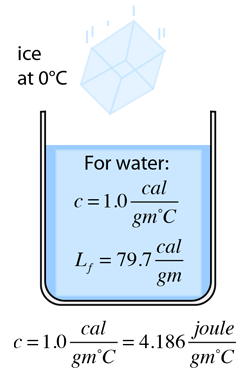# Cooling With Ice

The cooling of a drink with a cube of ice is more effective than using cold water because of the energy extracted from the drink to melt the ice (heat of fusion). The cooling of a container of water with a mass of ice at 0 C illustrates the energy of the phase change and the approach to thermal equilibrium.Ice added Water container Mass of ice = gm Mass of water = gm Initial water temperature = °C
Qgained by ice = Qlost by water
miLf + cmi(Tf - 0°C) = cmw(0°C -Tf)
 cal + Tf cal = cal - Tf cal
 Final temperature Tf = °C

The masses and initial water temperature may be changed. The calculation in the form above assumes that all the ice melts. If that is not the case, then the expression above would give a negative temperature which would of course be incorrect because the specific heat expressions used do not apply when a phase change is reached. If conditions are such that not all the ice melts, then the final temperature will be 0°C and some of the ice will remain unmelted.

Ice remaining unmelted = gm.

Index

Heat transfer concepts

Heat transfer examples

Thermal equilibrium

 HyperPhysics***** Thermodynamics R Nave
Go Back